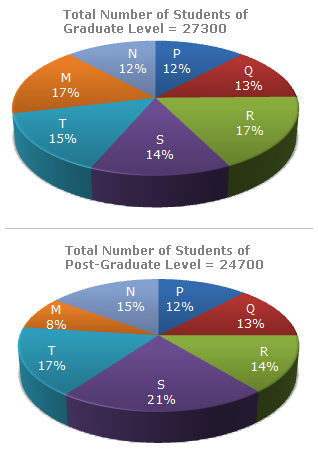# Data Interpretation - Pie Charts

Directions to Solve

The following pie-charts show the distribution of students of graduate and post-graduate levels in seven different institutes in a town.1.
What is the total number of graduate and post-graduate level students is institute R?
8320
7916
9116
8099
Explanation:

 Required number = (17% of 27300) + (14% of 24700) = 4641 + 3458 = 8099.

2.
What is the ratio between the number of students studying at post-graduate and graduate levels respectively from institute S?
14 : 19
19 : 21
17 : 21
19 : 14
Explanation:

 Required ratio = (21% of 24700) = (21 x 24700) = 19 . (14% of 27300) 14 x 27300 14

3.
How many students of institutes of M and S are studying at graduate level?
7516
8463
9127
9404
Explanation:

Students of institute M at graduate level= 17% of 27300 = 4641.

Students of institute S at graduate level = 14% of 27300 = 3822.Total number of students at graduate in institutes M and S = (4641 + 3822) = 8463.

4.
What is the ratio between the number of students studying at post-graduate level from institutes S and the number of students studying at graduate level from institute Q?
13 : 19
21 : 13
13 : 8
19 : 13
Explanation:

 Required ratio = (21% of 24700) = (21 x 24700) = 19 . (13% of 27300) 13 x 27300 13

5.
Total number of students studying at post-graduate level from institutes N and P is
5601
5944
6669
8372